# PDA Python DataFrames and Graphs Webinar 2020

Last updated: April 23rd, 2020

# Python DataFrames and Basic Graphs¶

Python is a popular programming language that can be used for analysis and graphing of large datasets. There are a lot of free resouces online to learn python:

In this short walk-through we are going to focus on practical Python packages for data analysis called Pandas and Seaborn. Packages add functionality to basic Python. Here are links to the documentation for these packages:

In Part 1 we will learn how to import csv files (comma-seperated values files) containing data and convert them into data frames. We'll see how to manipulate the data frames and perform summary statistics.

In Part 2 we will use Seaborn to make several basic, yet exciting, graph types from the data.

These commands will import the Python packages we are using:

In :
#import numpy, a numerical python package that allows fast analysis of data in matrices
import numpy as np
#import pandas, a python package that introduces data frames and requires numpy
import pandas as pd
#import matplotlib.pyplot, a python package for making graphs
import matplotlib.pyplot as plt
#import seaborn, a python package that can make nice scientific graphs and requires matplotlib
import seaborn as sns


This next command is so that the graphs will be plotted to the right size in the notebook. It only works in Jupyter Notebooks.

In :
%matplotlib inline


## Part 1: Creating the data frame and summary statistics¶

We are going to use a built-in dataset from the seaborn package called 'mpg' that includes data on different types of cars. This dataset can be accessed directly from seaborn, but we are going to save it to a csv (comma seperated variable) file and reopen it as a csv to demonstrate importing files with python.

In :
#accessing the built-in mpg dataset and saving it as the variable 'df'

#saving 'df' as a file called 'mpg.csv'. You can also use a longer file path to save to a different location.
df.to_csv('mpg.csv', index = False)

In :
#reading the 'mpg.csv' file


Try checking all the read_csv function options by hitting 'shift + tab' while you are typing inside the parenthesis! This works for all python functions in Jupyter Notebooks.

In :
#display the df
df

Out:
mpg cylinders displacement horsepower weight acceleration model_year origin name
0 18.0 8 307.0 130.0 3504 12.0 70 usa chevrolet chevelle malibu
1 15.0 8 350.0 165.0 3693 11.5 70 usa buick skylark 320
2 18.0 8 318.0 150.0 3436 11.0 70 usa plymouth satellite
3 16.0 8 304.0 150.0 3433 12.0 70 usa amc rebel sst
4 17.0 8 302.0 140.0 3449 10.5 70 usa ford torino
... ... ... ... ... ... ... ... ... ...
393 27.0 4 140.0 86.0 2790 15.6 82 usa ford mustang gl
394 44.0 4 97.0 52.0 2130 24.6 82 europe vw pickup
395 32.0 4 135.0 84.0 2295 11.6 82 usa dodge rampage
396 28.0 4 120.0 79.0 2625 18.6 82 usa ford ranger
397 31.0 4 119.0 82.0 2720 19.4 82 usa chevy s-10

398 rows × 9 columns

You can select and view specific columns or rows:

In :
#selecting a specific column of the data frame
df['weight']

Out:
0      3504
1      3693
2      3436
3      3433
4      3449
...
393    2790
394    2130
395    2295
396    2625
397    2720
Name: weight, Length: 398, dtype: int64
In :
#selecting a specific row of the data frame
#note when using iloc that numbering starts at 0 not 1 for both rows and columns
df.iloc[396 , : ]

Out:
mpg                      28
cylinders                 4
displacement            120
horsepower               79
weight                 2625
acceleration           18.6
model_year               82
origin                  usa
name            ford ranger
Name: 396, dtype: object

To select multiple rows or columns you can use the ":" symbol. For example:

df.iloc[1 , 5] gives the second row and the fourth column.

df.iloc[0:2 , 5] gives the first 2 rows and forth column.

The ":" symbol used alone means ALL columns or ALL rows.

In :
df.iloc[0:2 , 5]

Out:
0    12.0
1    11.5
Name: acceleration, dtype: float64

Note that the index is just a list of numbers, and it would be more useful to use the column "name" as the index. You can change the index as follows:

In :
#making one of the columns into the index of the dataframe
df = df.set_index('name')

df

Out:
mpg cylinders displacement horsepower weight acceleration model_year origin
name
chevrolet chevelle malibu 18.0 8 307.0 130.0 3504 12.0 70 usa
buick skylark 320 15.0 8 350.0 165.0 3693 11.5 70 usa
plymouth satellite 18.0 8 318.0 150.0 3436 11.0 70 usa
amc rebel sst 16.0 8 304.0 150.0 3433 12.0 70 usa
ford torino 17.0 8 302.0 140.0 3449 10.5 70 usa
... ... ... ... ... ... ... ... ...
ford mustang gl 27.0 4 140.0 86.0 2790 15.6 82 usa
vw pickup 44.0 4 97.0 52.0 2130 24.6 82 europe
dodge rampage 32.0 4 135.0 84.0 2295 11.6 82 usa
ford ranger 28.0 4 120.0 79.0 2625 18.6 82 usa
chevy s-10 31.0 4 119.0 82.0 2720 19.4 82 usa

398 rows × 8 columns

Now we can select rows by name of vehicle:

In :
#selcting a specific row by name
df.loc['buick skylark 320', : ]

#note the method is loc, not iloc now

Out:
mpg               15
cylinders          8
displacement     350
horsepower       165
weight          3693
acceleration    11.5
model_year        70
origin           usa
Name: buick skylark 320, dtype: object

To delete rows use the 'drop' function

An important thing to note is that any function which modifies the data by default will generate a copy of the data frame, and not change the data frame itself. This is prevent accidental errors. To apply changes to the data frame itself the option "inplace=True" needs to be included.

In :
#deleting a column (does not modify df permenantly, but allows you to see result)
df.drop('origin', axis = 1)

Out:
mpg cylinders displacement horsepower weight acceleration model_year
name
chevrolet chevelle malibu 18.0 8 307.0 130.0 3504 12.0 70
buick skylark 320 15.0 8 350.0 165.0 3693 11.5 70
plymouth satellite 18.0 8 318.0 150.0 3436 11.0 70
amc rebel sst 16.0 8 304.0 150.0 3433 12.0 70
ford torino 17.0 8 302.0 140.0 3449 10.5 70
... ... ... ... ... ... ... ...
ford mustang gl 27.0 4 140.0 86.0 2790 15.6 82
vw pickup 44.0 4 97.0 52.0 2130 24.6 82
dodge rampage 32.0 4 135.0 84.0 2295 11.6 82
ford ranger 28.0 4 120.0 79.0 2625 18.6 82
chevy s-10 31.0 4 119.0 82.0 2720 19.4 82

398 rows × 7 columns

In :
#note that our original data frame still includes 'name' column!
df

Out:
mpg cylinders displacement horsepower weight acceleration model_year origin
name
chevrolet chevelle malibu 18.0 8 307.0 130.0 3504 12.0 70 usa
buick skylark 320 15.0 8 350.0 165.0 3693 11.5 70 usa
plymouth satellite 18.0 8 318.0 150.0 3436 11.0 70 usa
amc rebel sst 16.0 8 304.0 150.0 3433 12.0 70 usa
ford torino 17.0 8 302.0 140.0 3449 10.5 70 usa
... ... ... ... ... ... ... ... ...
ford mustang gl 27.0 4 140.0 86.0 2790 15.6 82 usa
vw pickup 44.0 4 97.0 52.0 2130 24.6 82 europe
dodge rampage 32.0 4 135.0 84.0 2295 11.6 82 usa
ford ranger 28.0 4 120.0 79.0 2625 18.6 82 usa
chevy s-10 31.0 4 119.0 82.0 2720 19.4 82 usa

398 rows × 8 columns

In :
#deleting a row in the original data frame
df.drop('buick skylark 320', axis = 0, inplace = True)

#not that by using inplace=True the original data frame is now permenantly changed!
df

Out:
mpg cylinders displacement horsepower weight acceleration model_year origin
name
chevrolet chevelle malibu 18.0 8 307.0 130.0 3504 12.0 70 usa
plymouth satellite 18.0 8 318.0 150.0 3436 11.0 70 usa
amc rebel sst 16.0 8 304.0 150.0 3433 12.0 70 usa
ford torino 17.0 8 302.0 140.0 3449 10.5 70 usa
ford galaxie 500 15.0 8 429.0 198.0 4341 10.0 70 usa
... ... ... ... ... ... ... ... ...
ford mustang gl 27.0 4 140.0 86.0 2790 15.6 82 usa
vw pickup 44.0 4 97.0 52.0 2130 24.6 82 europe
dodge rampage 32.0 4 135.0 84.0 2295 11.6 82 usa
ford ranger 28.0 4 120.0 79.0 2625 18.6 82 usa
chevy s-10 31.0 4 119.0 82.0 2720 19.4 82 usa

397 rows × 8 columns

New columns can be added to the dataframe as well. For example, if you wanted a normalized version of one of the column variables you could create a new column using mathematical operations on columns:

In :
#creating a new column
df['normalized displacement'] = (df['displacement'] - df['displacement'].mean()) / df['displacement'].std() * 100


Now lets look at some info about the data frame:

In :
df.info()

<class 'pandas.core.frame.DataFrame'>
Index: 397 entries, chevrolet chevelle malibu to chevy s-10
Data columns (total 9 columns):
#   Column                   Non-Null Count  Dtype
---  ------                   --------------  -----
0   mpg                      397 non-null    float64
1   cylinders                397 non-null    int64
2   displacement             397 non-null    float64
3   horsepower               391 non-null    float64
4   weight                   397 non-null    int64
5   acceleration             397 non-null    float64
6   model_year               397 non-null    int64
7   origin                   397 non-null    object
8   normalized displacement  397 non-null    float64
dtypes: float64(5), int64(3), object(1)
memory usage: 31.0+ KB


Note that the data types (dtypes) include float64, int64 and object. These were automatically interpreted by pandas when you loaded in the csv file! Numbers with decimals are dtype float64, numbers that are integers are dtype int64, and strings are stored as objects.

Also note that there are 6 null values in the 'horsepower' column, meaning some data is missing.

In :
#removing rows with missing data
df.dropna(inplace = True)


You can change the dtype of any column, although sometimes you will run into errors if there are still missing values. The dtyle 'category' is more versatile than 'object' for categorical variables when it comes to graphing.

In :
#change to category dtype
df['cylinders'] = df['cylinders'].astype('category')
df['orgin'] = df['origin'].astype('category')

#change to integer dtype (will round decimals)
df['displacement'] = df['displacement'].astype('int16')


To see the summary statistics for each numerical column you can use the describe method:

In :
df.describe()

Out:
mpg displacement horsepower weight acceleration model_year normalized displacement
count 391.000000 391.000000 391.000000 391.000000 391.000000 391.000000 391.000000
mean 23.467519 194.012788 104.314578 2975.754476 15.551662 75.994885 0.943847
std 7.803266 104.481883 38.418088 849.716985 2.754789 3.675975 100.362088
min 9.000000 68.000000 46.000000 1613.000000 8.000000 70.000000 -120.102770
25% 17.250000 105.000000 75.000000 2224.500000 13.800000 73.000000 -84.561303
50% 23.000000 151.000000 93.000000 2800.000000 15.500000 76.000000 -40.374613
75% 29.000000 264.500000 125.000000 3611.000000 17.050000 79.000000 68.651239
max 46.600000 455.000000 230.000000 5140.000000 24.800000 82.000000 251.641767

You can also calculate summary statistics by group for categorical variables!

In :
#creating the groups based off a categorical column
by_origin = df.groupby('origin')

#you can use any built-in statistics function on the groups
by_origin.mean()

Out:
mpg displacement horsepower weight acceleration model_year normalized displacement
origin
europe 27.602941 109.632353 80.558824 2433.470588 16.794118 75.676471 -80.111556
japan 30.450633 102.708861 79.835443 2221.227848 16.172152 77.443038 -86.762126
usa 20.054098 247.090164 118.860656 3371.176230 15.004508 75.614754 51.929664

Some other useful data representations are correlation tables and pivot tables:

In :
#creating a correlation matrix for the numerical variables
df.corr()

Out:
mpg displacement horsepower weight acceleration model_year normalized displacement
mpg 1.000000 -0.804483 -0.777698 -0.831913 0.421055 0.578863 -0.804490
displacement -0.804483 1.000000 0.896643 0.933281 -0.541239 -0.365923 1.000000
horsepower -0.777698 0.896643 1.000000 0.864672 -0.687359 -0.412518 0.896646
weight -0.831913 0.933281 0.864672 1.000000 -0.415196 -0.306931 0.933279
acceleration 0.421055 -0.541239 -0.687359 -0.415196 1.000000 0.285972 -0.541239
model_year 0.578863 -0.365923 -0.412518 -0.306931 0.285972 1.000000 -0.365940
normalized displacement -0.804490 1.000000 0.896646 0.933279 -0.541239 -0.365940 1.000000
In :
#creating a pivot table (reorganization of data)
df.pivot_table(index = "origin", columns = "model_year", values = "mpg")

Out:
model_year 70 71 72 73 74 75 76 77 78 79 80 81 82
origin
europe 25.200000 28.750000 22.000000 24.000000 27.000000 24.50 24.250000 29.250000 24.950000 30.450000 36.8375 30.600000 40.000000
japan 25.500000 29.500000 24.200000 20.000000 29.333333 27.50 28.000000 27.416667 29.687500 32.950000 35.4000 32.958333 34.888889
usa 15.285714 17.736842 16.277778 15.034483 18.142857 17.55 19.431818 20.722222 21.772727 23.478261 26.3000 27.530769 29.789474

## Part 2:¶

### Graphing with Seaborn¶

First we can set some basic plot style parameters with sns.set

If you ever want to know more about what the options in a function are you can check it's documentation page by searching online, or you can hit 'shift + tab' when you are typing inside it's parenthesis in Jupyter notebook!

In :
sns.set(context = 'notebook', style = 'ticks', palette = 'muted', font = 'sans-serif', font_scale = 1.5)


You can also use the matplotlib.rcParams function to modify a lot of plot features.

#### Bar Plots¶

These are good to use when plotting categorical data and continous data.

The default error bars is 95% confidence interval (bootstrapped) in Seaborn.

You can change this by setting the option ci = 90 for 90% confidence interval, or ci = 'sd' for standard deviation

In :
sns.barplot(x = 'cylinders', y = "weight", ci = 'sd', data = df)

Out:
<matplotlib.axes._subplots.AxesSubplot at 0x7f6529d7c790>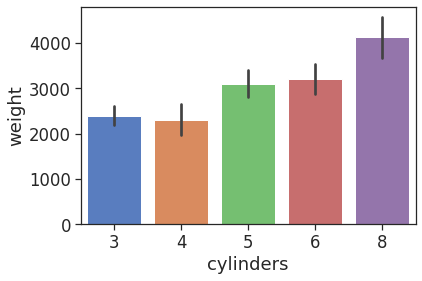Changing the x and y variables can makes the plot horizontal instead of vertical.

You can also adjust the order of the bars using the order option, and passing it a list of the categories in the order you want.

In :
sns.barplot(x = 'weight', y = 'cylinders', data = df, order = [8,6,5,4,3])

Out:
<matplotlib.axes._subplots.AxesSubplot at 0x7f6529d25880>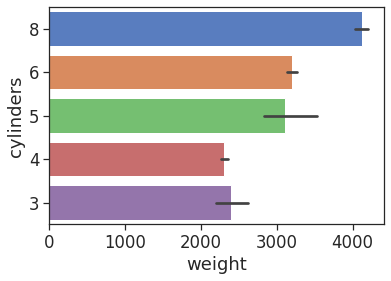### Changing plot aesthetics¶

You can have a lot of control over plot aesthetics in Python, however, I found that learning how to change aesthetics was challenging, and was sometimes different for each plot type. The best way to learn is using the package documentation and also by searching for what you want online, there are lot of tutorials.

Note that you can use commands from Matplotlib to change aesthetics in Seaborn plots too, because Seaborn is built on top of Matplotlib.

Below I show how to make some stylistic changes to bar plots:

In :
#change plot size and dimensions (inches (width,height))
#You must run this command first
plt.figure(figsize=(10,5))

#adding error bar caps, changing the error bar color and width, and adding bar edges
sns.barplot(x = 'cylinders', y = 'weight', data = df, capsize = 0.2, errcolor = '0', edgecolor = '0',errwidth = '1')

#remove the right and top edges of the plot
sns.despine()

#rotate the x-axis labels 45 degrees
plt.xticks(rotation = 45)

#change y axis scale
plt.yscale("log")

#set y axis tick marks
plt.yticks([2000, 3000, 4000, 5000])

plt.xlabel("Number of Cylinders")
plt.ylabel("Weight (lbs)")
plt.title("Car Weight Increases with Cylinders")

Out:
Text(0.5, 1.0, 'Car Weight Increases with Cylinders')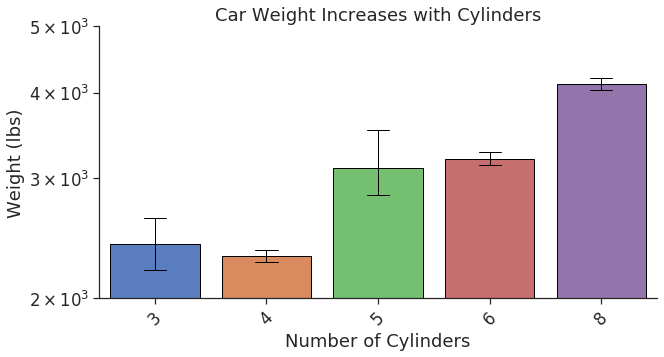### Box Plots¶

The box is the interquartile range (contains 50% of the data). The center line is the median. The whiskers can be modified with the "whis" option, the default is whis = 1.5, which is the "Proportion of the IQR past the low and high quartiles to extend the plot whiskers".

In :
sns.boxplot(x = 'model_year', y = 'mpg', data = df)

Out:
<matplotlib.axes._subplots.AxesSubplot at 0x7f65296eedf0>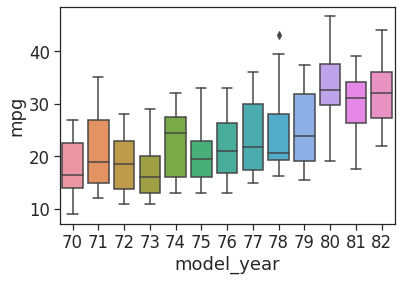In :
sns.boxplot(x = 'model_year', y = 'mpg', data = df, notch = True)

Out:
<matplotlib.axes._subplots.AxesSubplot at 0x7f65295295b0>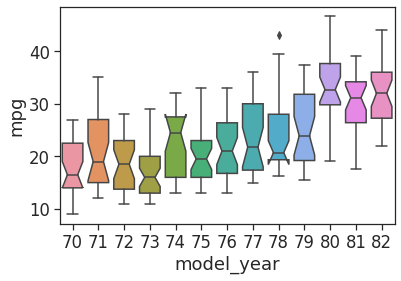### Cat Plot¶

This is one way you can add a second category variable to the plot (the 'hue' option).

By specifying 'kind' you can make a 'bar' plot or others.

In :
sns.catplot(x = 'cylinders', y = "mpg", hue = "origin", data = df, kind = 'box')

Out:
<seaborn.axisgrid.FacetGrid at 0x7f65293f9640>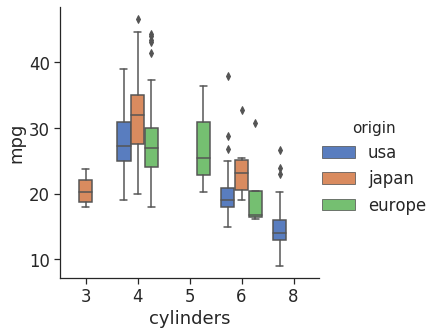In :
sns.catplot(x = 'cylinders', y = "mpg", hue = "origin", data = df, kind = 'swarm')

Out:
<seaborn.axisgrid.FacetGrid at 0x7f65272f42e0>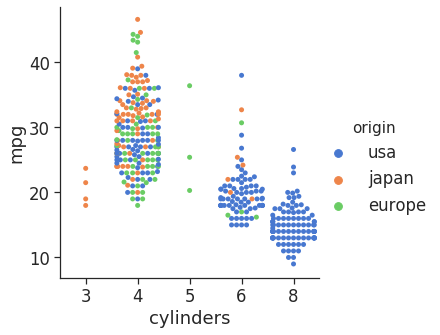#### Distplot¶

Useful to check the distribution of a continous variable.

This is a histogram with a KDE (kernal density estimate) overlayed on top of it.

In :
sns.distplot(df['horsepower'])

Out:
<matplotlib.axes._subplots.AxesSubplot at 0x7f65272a31c0>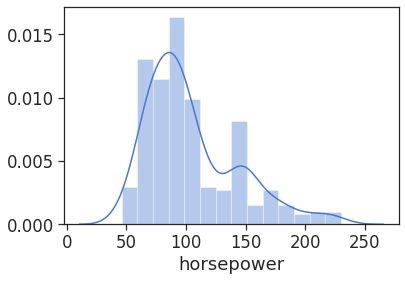It is possible to change the number of bins and remove the KDE line:

In :
sns.distplot(df['horsepower'], bins = 5,kde = False, color = "black")

Out:
<matplotlib.axes._subplots.AxesSubplot at 0x7f652720e700>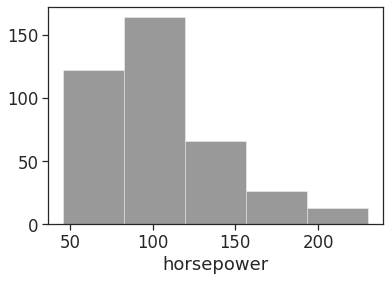#### Lineplot¶

Good for plotting a continous variable against an ordered variable like 'model_year'

This automatically includes a 95% confidence interval that you can change to standard deviation using ci="sd" similarly to for barplots earlier.

In :
sns.lineplot(x = "model_year", y = "horsepower", data = df)

Out:
<matplotlib.axes._subplots.AxesSubplot at 0x7f65271ec040>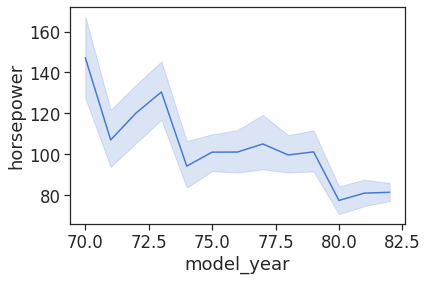In :
#style='origin' makes different style lines for each origin
#err_style='bars' converts to using bars to represent error
sns.lineplot(x = "model_year", y = "horsepower", style = "origin", err_style = "bars", color = 'black', data = df)

#choose legend location
plt.legend(loc = "upper right", fontsize = "small")

Out:
<matplotlib.legend.Legend at 0x7f6527110910>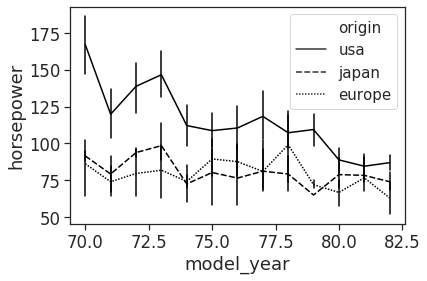In :
#hue='origin' uses a different color line for each origin
sns.lineplot(x = 'model_year', y = 'mpg', hue = "origin", data = df)

#choose legend location, and place legend outside graph. bbox_to_anchor moves legend from starting position.
plt.legend(loc = "center left", bbox_to_anchor = (1,0.5))

Out:
<matplotlib.legend.Legend at 0x7f6527090af0>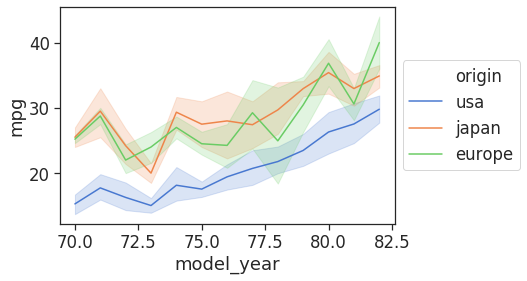#### Scatterplots¶

Good for plotting two continuous variables

In :
sns.scatterplot(x = 'horsepower', y = 'displacement', data = df)

Out:
<matplotlib.axes._subplots.AxesSubplot at 0x7f65270c3f40>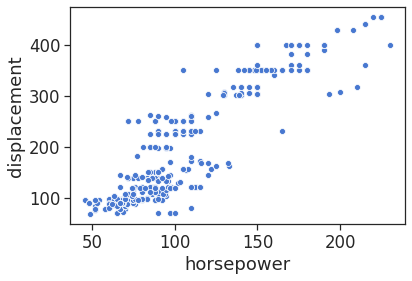In :
#the alpha option determines how transparent the dots are, so you can see where they pile up
sns.scatterplot(x = 'horsepower', y = 'displacement', data = df, alpha = 0.5, color = 'black')

Out:
<matplotlib.axes._subplots.AxesSubplot at 0x7f6527025b20>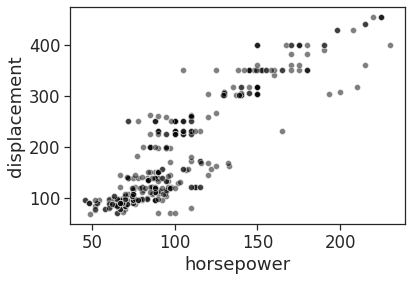In :
sns.scatterplot(x = 'horsepower', y = "displacement", hue = "origin", data = df)

plt.legend(loc = "center left", bbox_to_anchor = (1,0.5), fontsize="x-small")

Out:
<matplotlib.legend.Legend at 0x7f6526fdaee0>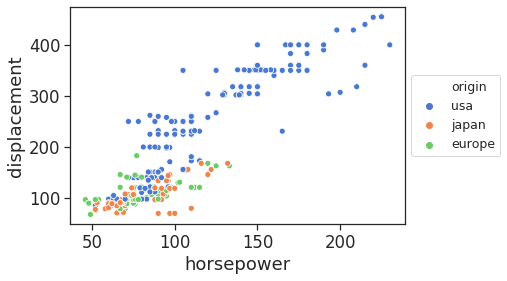#### Jointplot¶

Jointplot combines scatter plot and dist plot. You can change how the data is graphed using the 'kind' option.

In :
sns.jointplot(x = 'horsepower', y = 'displacement', data = df, kind = 'reg')

Out:
<seaborn.axisgrid.JointGrid at 0x7f6526f4e790>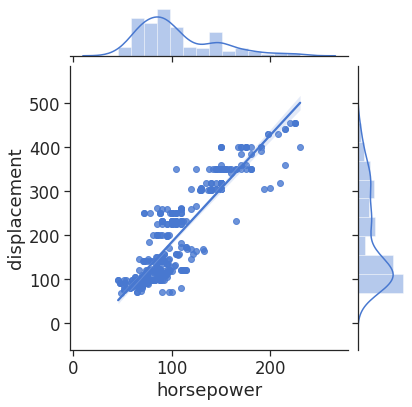In :
df["horsepower"].corr(df["displacement"],method="pearson")

#I couldn't figure out how to get the p-value for the correlation, if anyone knows, let me know!

Out:
0.8966434470554115

#### Heatmap plots¶

In :
pt = df.pivot_table(index = "origin", columns = "model_year", values = "mpg")
sns.heatmap(pt, cmap = 'coolwarm')

Out:
<matplotlib.axes._subplots.AxesSubplot at 0x7f6526e60670>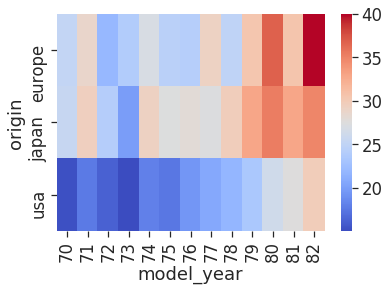#### Pairplots¶

These are a great way to explore your data. The diagonal shows the distribution of the data for each variable, and the sides show scatterplots of variables plotted against eachother. You can use the 'hue' option to have different colors by an additional variable.

Here I used only a subset of 'df' because there were initally too many columns. I used df.iloc[:,[0,4,5,7]] to specify all the rows (:) and a list of columns ([0,4,5,7]). Remember that the numbering begins at 0 in python!

In :
sns.pairplot(data = df.iloc[:,[0,4,5,7]], hue = 'origin')

Out:
<seaborn.axisgrid.PairGrid at 0x7f6526df7a00>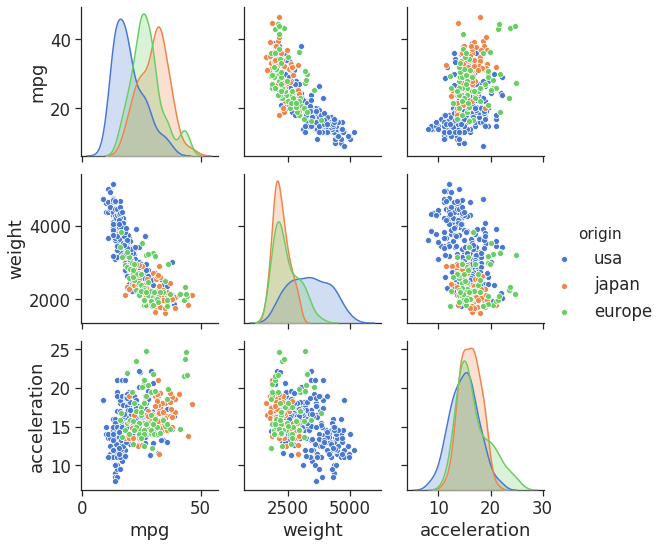### Saving a plot¶

You can save a plot as any image type just by adding .jpg, .tiff, .png, ect in the file name. The image resolution can be set using the 'dpi' option. Note that you can include a longer file path to save to a specific location, otherwise it will save to your current working directory.

In :
my_plot = sns.pairplot(data = df.iloc[:,[0,4,5,7]], hue = 'origin')

my_plot.savefig('my_plot.tiff', dpi = 300)

#pdf save option where text is editable in programs such as Illustrator:
plt.rcParams['pdf.fonttype'] = 42

my_plot.savefig('filename.pdf')If you can't find your saved plot, you can check your current working directory using the following:

In :
import os

print(os.getcwd())

/app

In [ ]:


In [ ]: